﻿ Some Common Fixed Point Theorems for Two Pairs of Weak Compatible Mappings of Type (A) in <i>G</i><SUB><i>b</i></SUB>-metric SpacePublications are Open
Access in this journal
Article Versions
Export Article
• Normal Style
• MLA Style
• APA Style
• Chicago Style
Research Article
Open Access Peer-reviewed

### Some Common Fixed Point Theorems for Two Pairs of Weak Compatible Mappings of Type (A) in Gb-metric Space

M. Koierng Meitei, Yumnam Rohen , R. S. Verma
American Journal of Applied Mathematics and Statistics. 2018, 6(4), 135-140. DOI: 10.12691/ajams-6-4-3
Published online: August 01, 2018

### Abstract

In this paper, we prove a common fixed point theorem for two pairs of weak compatible mappings of type (A) in Gb-metric space. Further our result is verified with the help of example.

### 1. Introduction and Preliminaries

Metric fixed point theory is one of the most important and fundamental area of analysis. Due to this a flood of research work have been generated from this area. As a part of this study generalisation of metric space becomes one of the most interesting topic in which many researchers have devoted and continued working. Since the introduction of metric space by Frachet, there is a lot of generalisation of this space. Some of them which can be mentioned are 2-metric space, D-metric space, cone metric space, fuzzy metric space, Menger space, probabilistic metric space, partial metric space, quasi metric space, b-metric space, multiplicative metric space, modular metric space, cyclic metric space, S-metric space, b-cone metric space etc.

In a recent paper, Aghajani et.al. 1 introduced a new generalisation of metric space. They used the concepts of both G-metric 2 and b-metric 3, 4, 5 and generated a new definition and named it as Gb-metric space. They also pointed out that the class of Gb-metric space is effectively larger than that of G-metric space and G-metric space becomes a particular case of Gb-metric space. They claimed that every Gb-metric space is topologically equivalent to a b-metric space. For more results on Gb-metric space one can study the research papers in 6, 7, 8, 9, 10 and references there in.

Definition 1.1 2

Let X be a nonempty set and G: X3 → R+ be a function satisfying the following properties:

1. G(x, y, z) = 0 if and only if x = y = z;

2. 0 < G(x, x, y) for all x, y X with x ≠ y;

3. G(x, x, y) ≤ G(x, y, z) for all x, y, z X with z ≠ y;

4. G(x, y, z) = G(x, z, y) = G(y, z, x) = . . .(symmetry in all three variables);

5. G(x, y, z) ≤ G(x, a, a) +G(a, y, z) for all x, y, z, a X (rectangle inequality).

Then the function G is called a G-metric on X and the pair (X, G) is called a G-metric space.

Following definition was given by I. A. Bakhtin 3

Definition 1.2 3

Let X be a (nonempty) set and b ≥ 1a given real number. A function d: X × X → R+ (nonnegative real numbers) is called a b-metric provided that, for all x, y, z X, the following conditions are satisfied:

1. d(x, y) = 0 if and only if x = y;

2. d(x, y) = d(y, x);

3. d(x, z) ≤ b[d(x, y) + d(y, z)]

The pair (X, d) is called a b-metric space with parameter b.

Definition 1.3 1

Let X be a nonempty set and b ≥ 1 be a given real number. Suppose that a mapping G: X × X × X → R+ satisfies:

(Gb1) G(x, y, z) = 0 if x = y = z,

(Gb2) 0 <G(x, x, y) for all x, y ∈ X with x, y,

(Gb3) G(x, x, y) ≤ G(x, y, z) for all x, y, z ∈ X with y ≠ z.

(Gb4) G(x, y, z) = G(p[x, y, z]), where p is a permutation of x, y, z (symmetry),

(Gb5) G(x, y, z) ≤ b(G(x, a, a) + G(a, y, z)) for all x, y, z, a ∈ X (rectangle inequality).

Then G is called a generalized b-metric and pair (X, G) is called a generalized b-metric space or Gb-metric space.

It should be noted that, the class of Gb-metric spaces is effectively larger than that of G-metric spaces. Following example given by Aghajani 1 shows that a Gb-metric on X need not be a G-metric on X.

Example 1.4 1

Let (X, G) be a G-metric space, and G(x, y, z) = G(x, y, z)p, where p > 1 is a real number. Note that G is a Gb-metric with b = 2p-1.

Also in the above example, (X, G) is not necessarily a G-metric space. For example, let X = R and G-metric G be defined by G(x, y, z) =1/3(|x -y| + |y-z| + |x -z|), for all x, y, z R. Then G(x, y, z)2 = 1/9(|x-y|+|y-z|+|x-z|)2 is a Gb-metric on R with b = 22-1 = 2, but it is not a G-metric on R. To see this, let x = 3, y = 5, z = 7, a = 7/2 we get, G(3, 5, 7) = 64/9, G(3, 7/2, 7/2) = 1/9, G(7/2, 5, 7)= 49/9, so G(3, 5, 7) = 64/9 ≤50/9 = G(3, 7/2, 7/2) + G(7/2, 5, 7).

Following definitions and properties are given in Aghajani et. al. 1.

Definition 1.5 1

A Gb-metric G is said to be symmetric if G(x, y, y) = G(y, x, x) for all x, y X.

Definition 1.6 1

Let (X, G) be a Gb-metric space then for x0X, r > 0, the Gb-ball with centre x0 and radius r is BG(x0, r) = {y X|G(x0, y, y)<r}.

For example, let X = R and consider the Gb-metric G defined by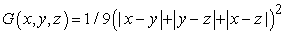for all x, y, z R. Then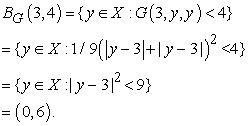By some straight forward calculations, we can establish the following.

Proposition 1.7 1

Let X be a Gb-metric space, then for each x, y, z, a X it follows that:

(1) if G(x, y, z) = 0 then x = y = z,

(2) G(x, y, z) ≤ b(G(x, x, y) + G(x, x, z)),

(3) G(x, y, y)≤ 2bG(y, x, x),

(4) G(x, y, z)≤b(G(x, a, z) + G(a, y, z))

Definition 1.8 1

Let X be a Gb-metric space, we define dG(x, y) = G(x, y, y)+G(x, x, y), it is easy to see that dG defines a b-metric on X, which we call it b-metric associated with G.

Proposition 1.9 1

Let X be a Gb-metric space, then for any x0 X and r > 0, if y BG(x0, r) then there exists a δ > 0 such that BG(y, δ) BG(x0, r).

Definition 1.10 1

Let X be a Gb-metric space. A sequence {xn} in X is said to be:

1. Gb-Cauchy sequence if, for each ε >0, there exists a positive integer n0 such that, for all m, n, l ≥ n0, G(xn, xm, xl) < ε;

2. Gb-convergent to a point x X if, for each ε > 0, there exists a positive integer n0 such that, for all m, n ≥ n0, G(xn, xm, x) < ε.

Proposition 1.11 1 Let (X, G) be a Gb-metric space, then the following are equivalent:

1. the sequence {xn} is Gb-Cauchy.

2. for any ε > 0, there exists n0 N such that G(xn, xm, xm) < ε, for all m, n ≥ n0.

Proposition 1.12 1 Let (X, G) be a Gb-metric space, then following are equivalent:

1. {xn} is Gb-convergent to x.

2. G(xn, xn, x)→ 0 as n → ∞.

3. G(xn, x, x)→ 0 as n → ∞.

Definition 1.13 1 A Gb-metric space X is called Gb-complete if every Gb-Cauchy sequence is Gb-convergent in X.

Definition 1.14 1 Let (X, G) and (X, G') be two Gb-metric spaces. Then a function f : X → X' is Gb-continuous at a point x X if and only if it is Gb-sequentially continuous at x, that is, whenever {xn} is Gb-convergent to x,{f (xn)} is G'b-convergent to f (x).

Lemma 1.15 1 Let (X, G) be a Gb-metric space with b1, and suppose that {xn}, {yn} and {zn} are Gb-convergent to x, y and z respectively. Then we have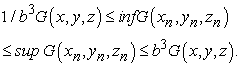In particular, if x = y = z, then we have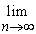G(xn,yn,zn) = ε.

Jungck 11 introduced the concept of compatible mappings in metric spaces. Jungck, Murthy and Cho 12 introduced the concept of compatible mappings of type (A) on metric spaces and proved some common fixed point theorems for compatible mappings of type (A). In 1995, Pathak, Kang and Beak 13 introduced the concept of weak compatible mapping of type (A) and proved some common fixed point theorems for weak compatible mappings of type (A) on Menger spaces. Readers can see about various forms of compatible mappings in the research papers in 14, 15, 16, 17, 18, 19, 20, 21, 22, 23, 24, 25, 26, 27, 28, 29, 30, 31, 32, 33 and references therein. We state the following definitions in the setting of Gb-metric space.

Definition 1.16 Let (X, G) be a Gb-metric space. A pair {f, g} is said to be compatible mappings if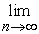G(fgxn, gfxn, gfxn)=0, whenever {xn} is a sequence in X such thatfxn=gxn=t for some t in X.

Definition 1.17 Let (X, G) be a Gb-metric space. A pair {f, g} is said to be compatible mappings of type (A) ifG(fgxn, ggxn, ggxn)=0 andG(gfxn, ffxn, ffxn)=0, whenever {xn} is a sequence in X such thatfxn=gxn=t for some t in X.

Definition 1.18 Let (X, G) be a Gb-metric space. A pair {f, g} is said to be weak compatible mappings of type (A) ifG(fgxn, ggxn, ggxn)=0, whenever {xn} is a sequence in X such thatfxn=gxn=t for some t in X.

The following propositions are easy to prove and hence we omit their proofs.

Proposition 1.19 Let f, g: (X, G)→(X, G) be mappings. If f and g are weak compatible mappings of type (A) and ft=gt for some t in X, then fgt=ggt.

Proposition 1.20 Let f, g: (X, G)→(X, G) be mappings. If f and g are weak compatible mappings of type (A) andfxn=gxn=t for some t in X. Then we havegfxn=ft, if f is continuous.

The aim of this paper is to prove a common fixed point theorem for two pairs of weak compatible mappings of type (A) in Gb-metric space.

### 2. Main Results

Our first result is the following common fixed point theorem.

Theorem 2.1 Suppose that f, g, M and T are self-mappings on a complete Gb-metric space (X, G) such that f(X) ⊆ T(X), g(X) ⊆ M(X). If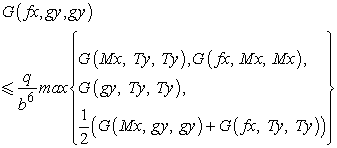holds for each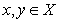with 0 < q <1 and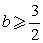, then f, g, M and T have a unique common fixed point in X provided that M and T are continuous and pairs {f, M} and{g, T}are compatible.

Proof. Let x0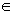X. As f(X) ⊆ T(X), there exists x1X such that fx0 = Tx1. Since gx1M(X), we can choose x2X such that gx1 = Mx2. In general, x2n+1 and x2n+2are chosen in X such that fx2n= Tx2n+1 and gx2n+1 = Mx2n+2. Define a sequence yn in X such that y2n= fx2n= Tx2n+1, and y2n+1 = gx2n+1 = Mx2n+2, for all n ≥ 0. Now, we show that yn is a Cauchy sequence. Consider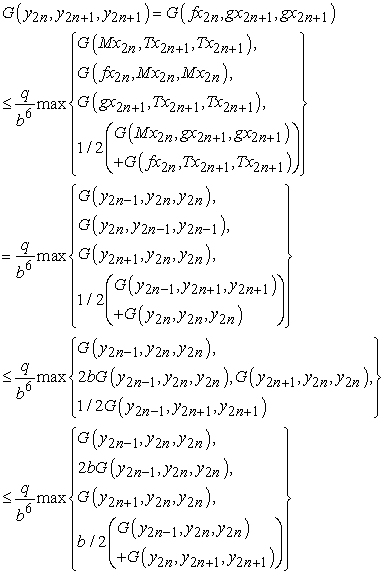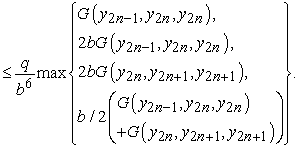Now, since G(y2n-1, y2n, y2n) ≤ 2bG(y2n-1, y2n, y2n) and G(y2n, y2n+1, y2n+1) ≤2bG(y2n, y2n+1, y2n+1) we have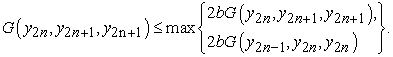If max = 2bG(y2n, y2n+1, y2n+1), we obtain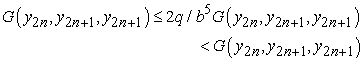So, max = 2bG(y2n-1, y2n, y2n) and we have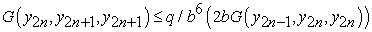i.e., G(y2n, y2n+1, y2n+1) ≤ 2q/b5G(y2n-1, y2n, y2n).

Let λ = 2q/b5. Since b ≥ 3/2 we have that 0 < λ <1.

Now,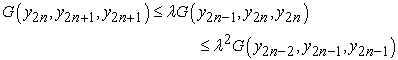and so on.

Hence, for all n ≥ 2, we obtain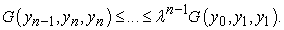(2)

Using (Gb5), and (2) for all n >m, we have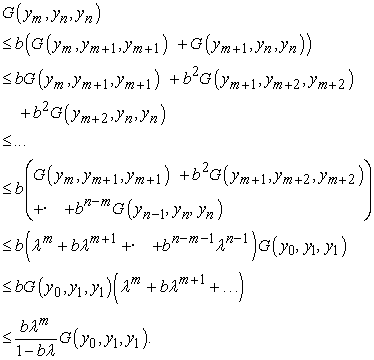On taking limit as m, n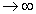, we have G(ym, yn, yn)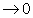as bλ<1. Therefore {yn} is a Cauchy sequence. Since X is a complete Gb-metric space, there is some y in X such that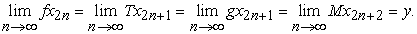We show that y is a common fixed point of f, g, M and T. Since M is continuous, therefore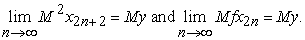Since the pair {f, M} is weak compatible type (A),G(fMx2n, Mfx2n, Mfx2n) = 0. So by proposition 1.20, we have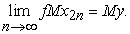Putting x = Mx2n and y = x2n+1 in (1) we obtain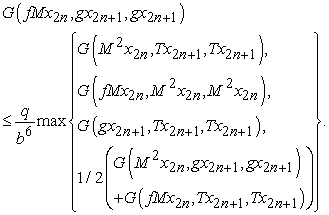(3)

Taking the upper limit as nin (3) and using Lemma 1.15, we get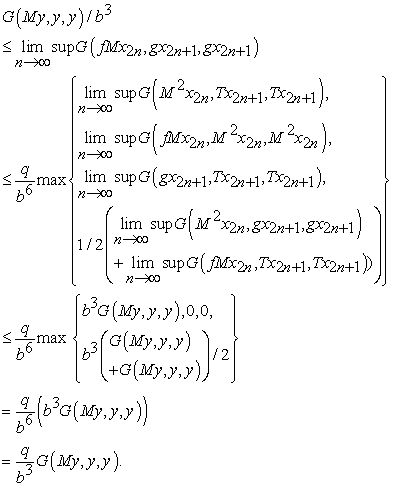Consequently, G(My, y, y) ≤ qG(My, y, y). As 0 < q <1, so My = y. Using continuity of T, we obtainT2x2n+1 = Ty andTgx2n+1 = Ty.

Since g and T are weak compatible type (A),G(gTxn, Tgxn, Tgxn) = 0. So, by proposition 1.20, we havegTx2n= Ty. Putting x = x2n and y = Tx2n+1 in (1), we obtain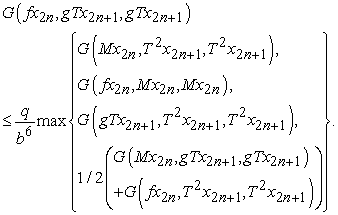(4)

Taking upper limit as nin (4) and using Lemma 1.15, we obtain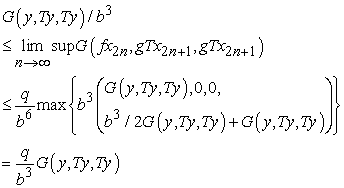which implies that Ty = y. Also, we can apply condition (1) to obtain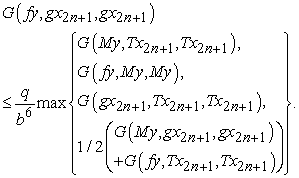(5)

Taking upper limit nin (5), and using My = Ty = y, we have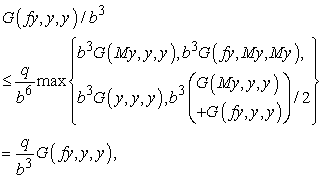which implies that G(fy, y, y) = 0 and fy= y as 0 < q <1. Finally, from condition (1), and the fact My = Ty = fy = y, we have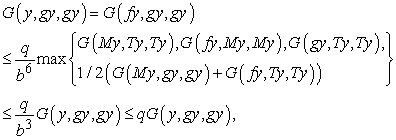which implies that G(y, gy, gy) = 0 and gy= y. Hence My = Ty = fy= gy= y. If there exists another common fixed point x in X for f, g, M and T, then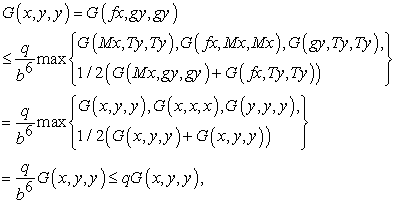which further implies that G(x, y, y) = 0 and hence, x = y. Thus, y is a unique common fixed point of f, g, M and T.

Example 2.2 Let X = [0, 1] be endowed with Gb-metric G∗(x, y, z) = (|y +z -2x|+|y- z|)2, where b = 4. Define f, g, M and T on X by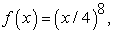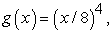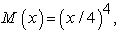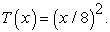Obviously, f(X) ⊆ T(X) and g(X) ⊆ M(X). Furthermore, the pairs {f, M} and {g, T} are weak compatible mappings of type (A). For each x, yX, we have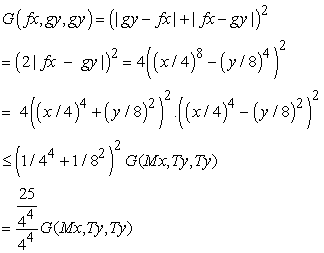where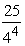q ≤ 1 and b = 4. Thus, f, g, M and T satisfy all condition of Theorem 2.1. Moreover 0 is the unique common fixed point of f, g, M and T.

Corollary 2.3 Let (X, G) be a complete Gb-metric space and f, g: X X two mappings such that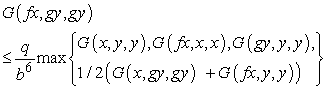holds for all x, yX with 0 < q <1 and b ≥ 3/2. Then, there exists a unique point y in X such that fy= gy = y.

Proof. If we take M = T = IX (identity mapping on X), then theorem 2.1 gives that f and g have a unique common fixed point.

Note. If we take f and g as identity maps on X, then Theorem 2.1 gives that M and T have a unique common fixed point.

Corollary 2.4 Let (X, G) be a complete Gb-metric space and f: X X mapping such that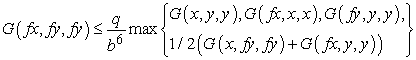holds for all x, yX with 0 < q <1 and b ≥ 3/2. Then f has a unique fixed point in X.

Proof. Take M and T as identity maps on X and f = g and then apply Theorem 2.1.

### Acknowledgments

Authors are thankful to the referees for their valuable suggestions.

### References

  Aghajani A., Abbas M. and Roshan J. R., Common Fixed Point of Generalized Weak Contractive Mappings in Partially Ordered Gb-metric Spaces, Filomat28:6 (2014), 1087-1101. In article View Article  Mustafa Z, Sims B. A new approach to generalized metric spaces. J Nonlinear Convex Anal, 2006, 7: 289297. In article View Article  I. A. Bakhtin, The contraction principle in quasimetric spaces, Funct. Anal., Gos. Ped. Inst. Unianowsk, 30(1989), 26-37. In article  Czerwik, S. Contraction mappings in b−metric spaces, Acta Math. Inf. Univ. Ostraviensis.1, (1993), 5-11. In article View Article  Czerwik, S. Nonlinear set-valued contraction mappings in b-metric spaces, Atti. Sem. Mat. Fis. Univ. Modena, 46(2), (1998), 263-276. In article View Article  Z. Mustafa, J.R. Roshan and V. Parvaneh, Coupled coincidence point results for (ψ, φ)-weakly contractive mappings in partially ordered Gb-metric spaces, Fixed Point Theory Appl., 2013:206 (2013). In article View Article  Z. Mustafa, J. R. Roshan and V. Parvaneh, Existence of a tripled coincidence point in ordered Gb-metric spaces and applications to a system of integral equations, Journal of Inequalities and Applications 2013, 2013:453. In article View Article  Shaban Sedghi, Nabi Shobkolaei, Jamal Rezaei Roshan and Wasfi Shatanawi, Coupled fixed point theorems in Gb-metric spaces, Matematiki Vesnik, 66, 2 (2014), 190-201 June 2014. In article View Article  Bulbul Khomdram, Yumnam Rohen and Thokchom Chhatrajit Singh, Coupled fixed point theorems in Gb-metric space satisfying some rational contractive conditions, SpringerPlus (2016), 5:1261. In article View Article  PubMed  Bulbul Khomdram and Yumnam Rohen, Quadrupled common fixed point theorems in Gb-metric spaces, IJPAM, 109(2), (2016), 279-293. In article  G. Jungck, Compatible mappings and common fixed points, Int. J. Math. Math. Sci., 9 (4) (1986), 771-779. In article View Article  Jungck, G., Murthy, P. P. and Cho, Y. J., Compatible mappings of type (A) and common fixed points, Math. Japonica, 38, (1993), 381-390. In article View Article  Pathak, H. K., Kang, S. M. and Baek, J. H., Weak compatible mappings of type (A) and common fixed point in Menger spaces, Comm. Korean Math. Soc., 10 (1), (1995), 67-83. In article View Article  Yumnam Rohen and Bidya Devi, Relationship between various types of compatible mappings, IJMSEA, 4(I) (2010), 67-82. In article View Article  Yumnam Rohen, Fixed point theorems of compatible mappings of type(R) in metric spaces, Int. J. Open Problems Compt. Math., 3(5), (2010) 138-143. In article View Article  L. Dwijendra and Yumnam Rohen, Common fixed point theorems of compatible mappings of type (A) in fuzzy metric spaces, IJMSEA, 5(VI) (2011), 307-316. In article View Article  M. Koireng and Yumnam Rohen, Common fixed point theorems of compatible mappings of type (P) in fuzzy metric spaces, Int. J. of Math. Anal., 6(4), (2012), 181-188. In article View Article  L. Ibeni and Yumnam Rohen, Related fixed point theorems for three set-valued mappings on three uniform spaces, Int. J. of Math. Anal., 6(9), (2012), 415-427 In article View Article  M. Krishnanado, Yumnam Rohen and L. Shambhu, Common fixed point theorems of compatible mappings of type(R), IJMSEA, 6(I) (2012), 355-361. In article View Article  Yumnam Rohen, Th. Indubala, O. Budhichandra and Leenthoi N., Common fixed point theorem for compatible mappings of type (A), IJMSEA, 6(II) (2012), 323-333. In article  M. Krishnanando, Yumnam Rohen, O. Budhichandra and Mairembam Bina, Weak compatible and compatible mappings of type (R) in cone metric space, IJMSEA, 6(II) (2012), 161-170. In article View Article  M. Koireng Meitei and Yumnam Rohen, Some fixed point theorems for compatible mappings in uniform space, IJMSEA, 6(IV) (2012), 107-115. In article  M. Koireng Meitei Leenthoi N. and Yumnam Rohen, Common fixed points of compatible mappings of type (R), General Mathematics Notes, 10(1) (2012), 58-62. In article View Article  M. Popeshwar Singh and Yumnam Rohen, Common fixed points of compatible mappings, Journal of Scientific Research, 4(3) (2012), 603-608. In article  L. Ibeni Devi and Yumnam Rohen, Compatible mappings of type (A-1) and type (A-2) in cone metric space, The Journal of Mathematics and Computer Sciences 2(6), (2012), 1612-1621. In article View Article  Yumnam Rohen and M. Ranjit Singh, Compatible mappings in cone metric space, for the book “Weakly commuting mappings after thirty years in metric fixed point theory” edited by S. Sessa, 2012 47-54. In article  Shahidur Rahman, Yumnam Rohen and M. Popeshwar Singh, Generalised common fixed point theorems of A-compatible and S-compatible mappings, American J. of Applied Mathematics and Statistics, 1(2), (2013), 27-29. In article View Article  L. Ibeni Devi and Yumnam Rohen, Remarks on common fixed point theorems of Rajesh et. al., IJMSEA, 7(VI) (2013) 97-109. In article  L. Bishwakumar and Yumnam Rohen, Common fixed point theorem for six self-maps in a cone metric space through semi compatibility and weak compatibility, IJMSEA, 7(VI) (2013) 121-133. In article  Yumnam Rohen and L. Premila Devi, Some fixed point theorems of semicompatible mappings, Journal of International Academy of Physical Sciences, 18(2) (2014) 125-129. In article View Article  Thokchom Chhatrajit and Yumnam Rohen, A comparative study of relationship among various types of spaces, International Journal of Applied Mathematics, 28(1) (2015), 29-36. In article View Article  Yumnam Rohen and L. Premila Devi, Some fixed point theorems of semi compatible and occasionally weakly compatible mappings in menger space, American Journal of Applied Mathematics and Statistics, 3(1) (2015), 29-33. In article View Article  Laishram Shanjit, Yumnam Rohen and P. P. Murthy, Some fixed point theorems of R-weakly commuting mappings in multiplicative metric spaces, Gazi University Journal of Science, 29(4) (2016) 855-867. In article View Article

Published with license by Science and Education Publishing, Copyright © 2018 M. Koierng Meitei, Yumnam Rohen and R. S. VermaThis work is licensed under a Creative Commons Attribution 4.0 International License. To view a copy of this license, visit http://creativecommons.org/licenses/by/4.0/